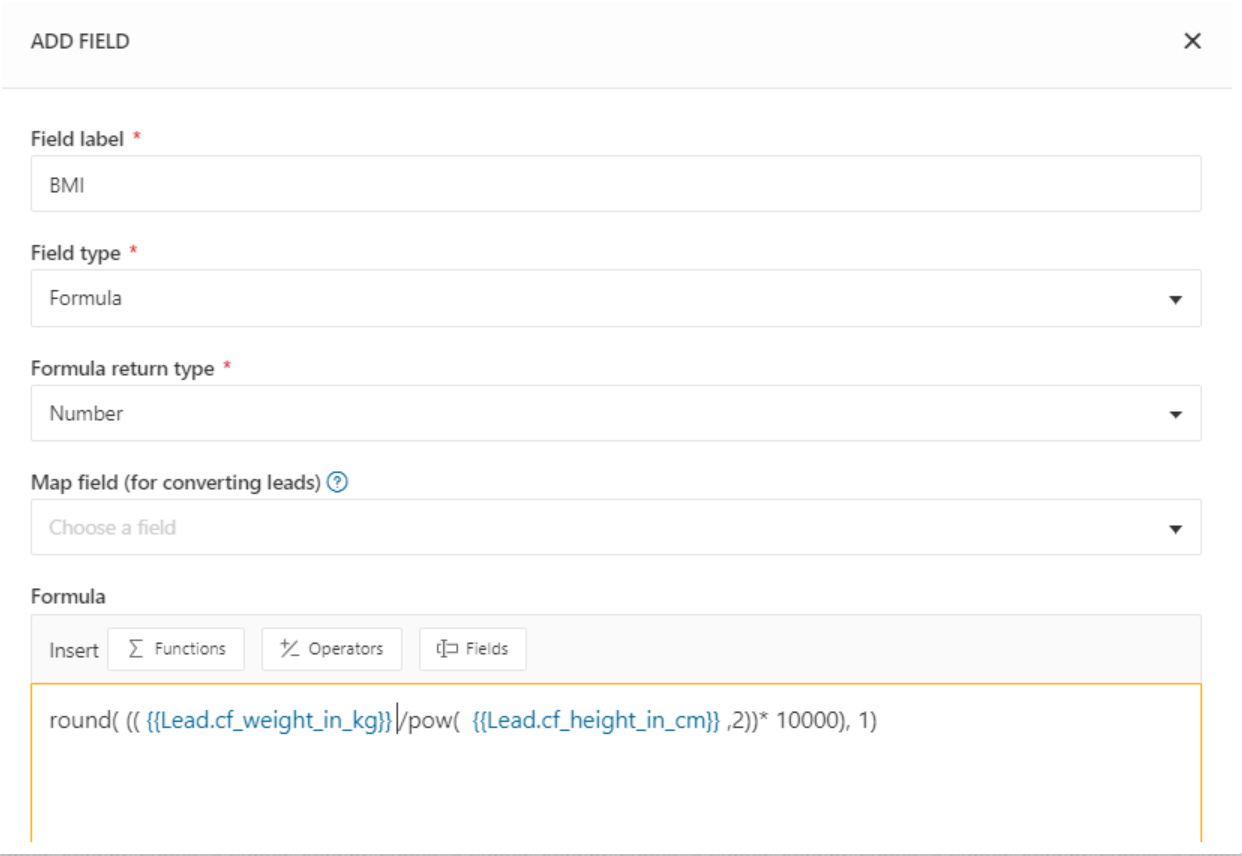## How do I configure a BMI calculator using formula fields? (Weight in kgs and Height in cms)?

#### Modified on: Thu, 23 Apr, 2020 at 11:23 AM

With the metric system, the formula for BMI is weight in kilograms divided by height in meters squared. Since we are using height in cm, we would have to divide weight (kg) by height (cm) squared, multiplied by 10,000 and round it to one decimal place.Did you find it helpful? Yes No

Start your 21-day free trial. No credit card required. No strings attached.

Start Free Trial

## How do I configure a BMI calculator using formula fields? (Weight in kgs and Height in cms)?

#### Modified on: Thu, 23 Apr, 2020 at 11:23 AM

With the metric system, the formula for BMI is weight in kilograms divided by height in meters squared. Since we are using height in cm, we would have to divide weight (kg) by height (cm) squared, multiplied by 10,000 and round it to one decimal place.Did you find it helpful? Yes No